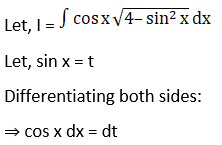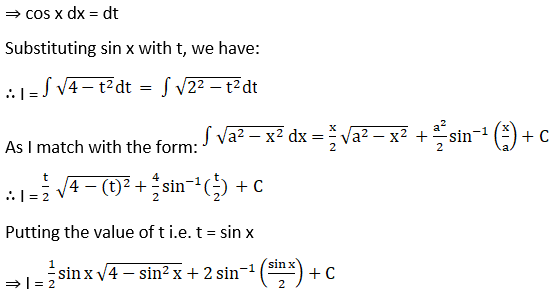# RD Sharma Solutions For Class 12 Maths Exercise 19.28 Chapter 19 Indefinite Integrals

In this exercise, we will prove the different formulae, which will be used in evaluating integrals of the form $\int \sqrt{ax^{2}+bx+c}\ dx$ and $\int (px+q) \sqrt{ax^{2}+bx+c}\ dx$ using integration by parts method. These solutions are formulated by BYJU’S experts after vast research on each topic to make learning easy for the students. The PDF of RD Sharma Solutions for Class 12 Maths Chapter 19 Indefinite Integrals Exercise 19.28 are provided here.

## Download the PDF of RD Sharma Solutions For Class 12 Chapter 19 – Indefinite Integrals Exercise 19.28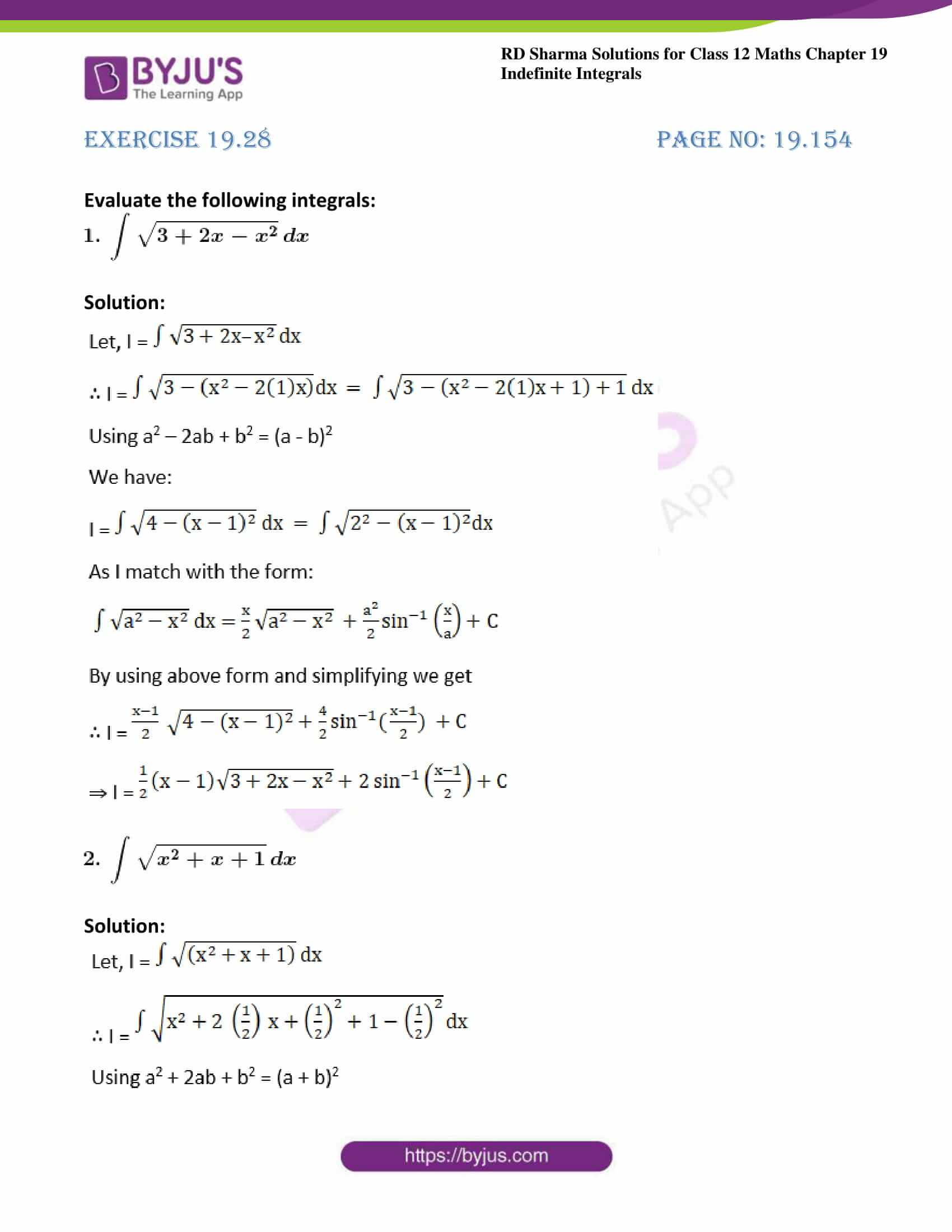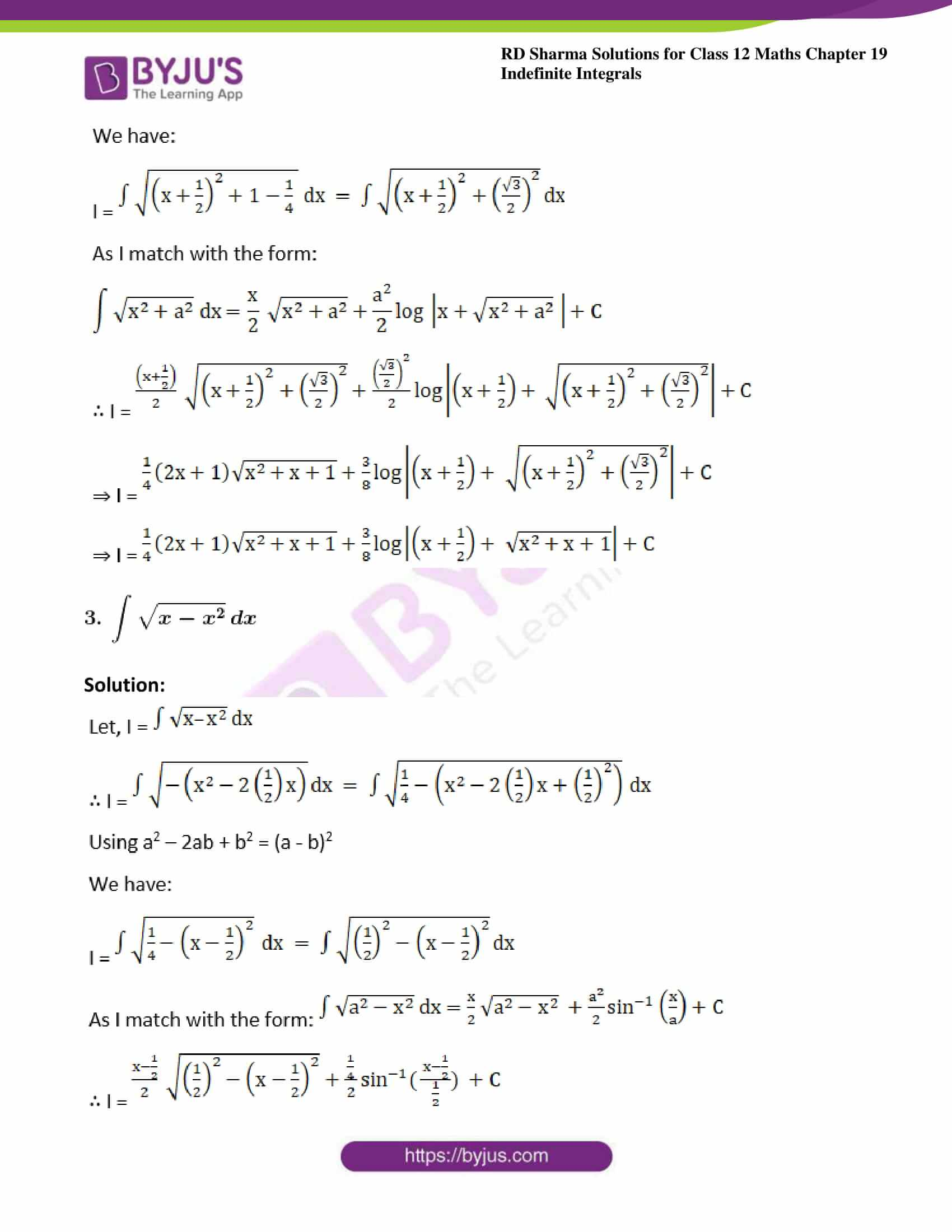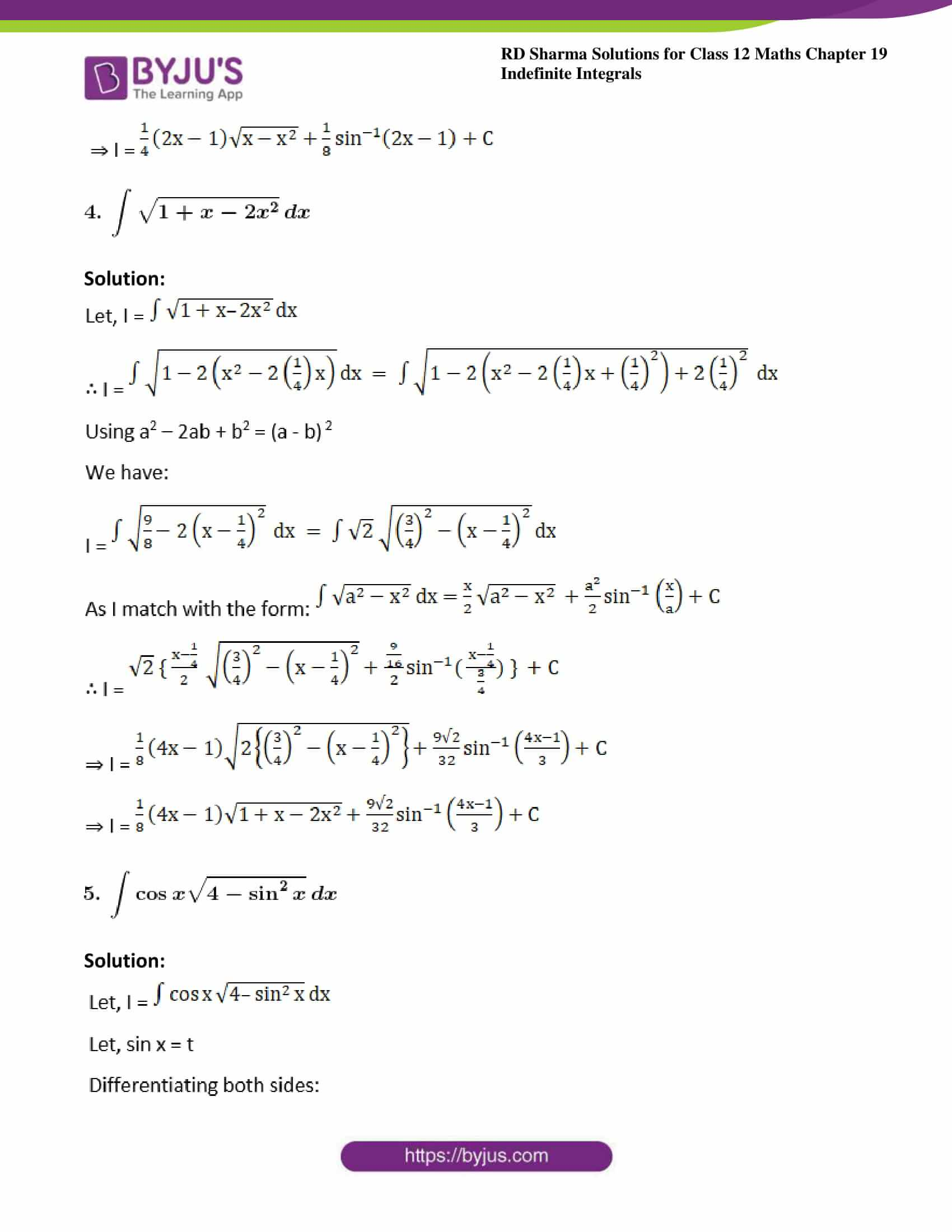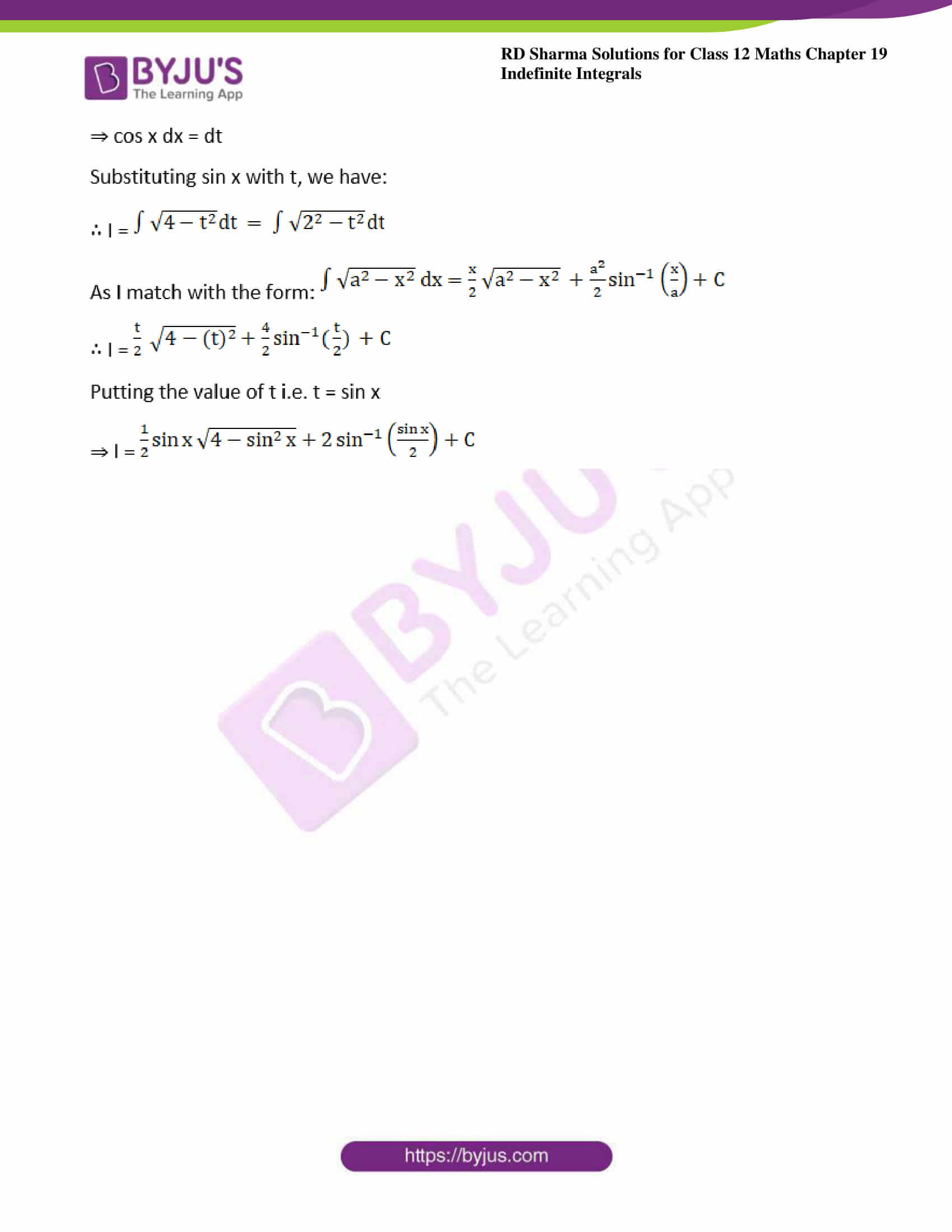### Access answers to Maths RD Sharma Solutions For Class 12 Chapter 19 – Indefinite Integrals Exercise 19.28

Evaluate the following integrals: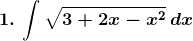Solution: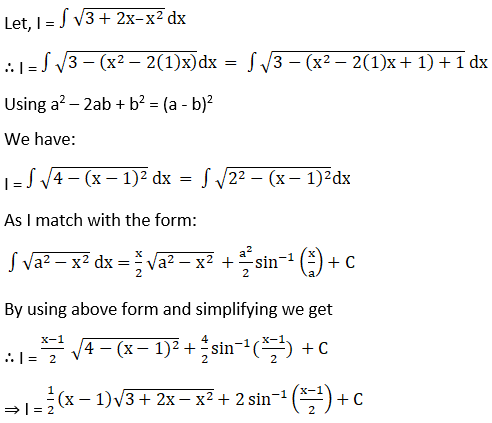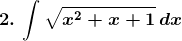Solution: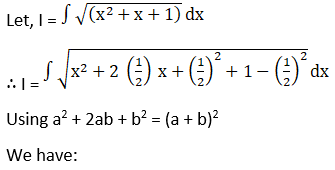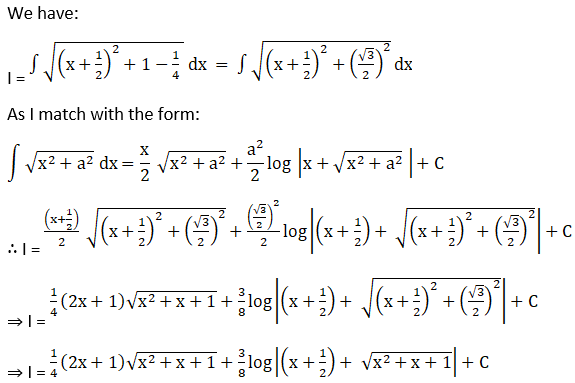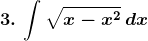Solution: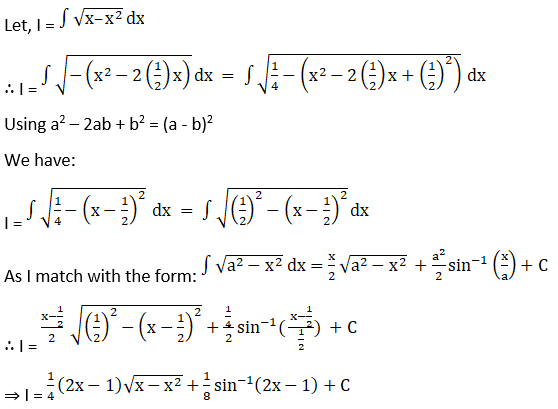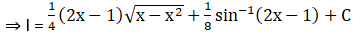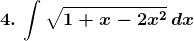Solution: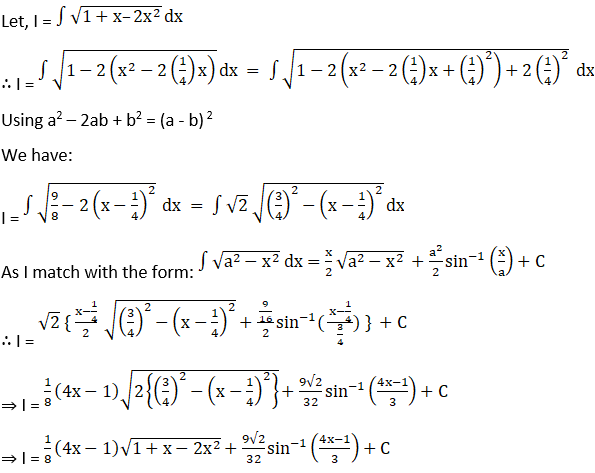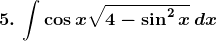Solution: